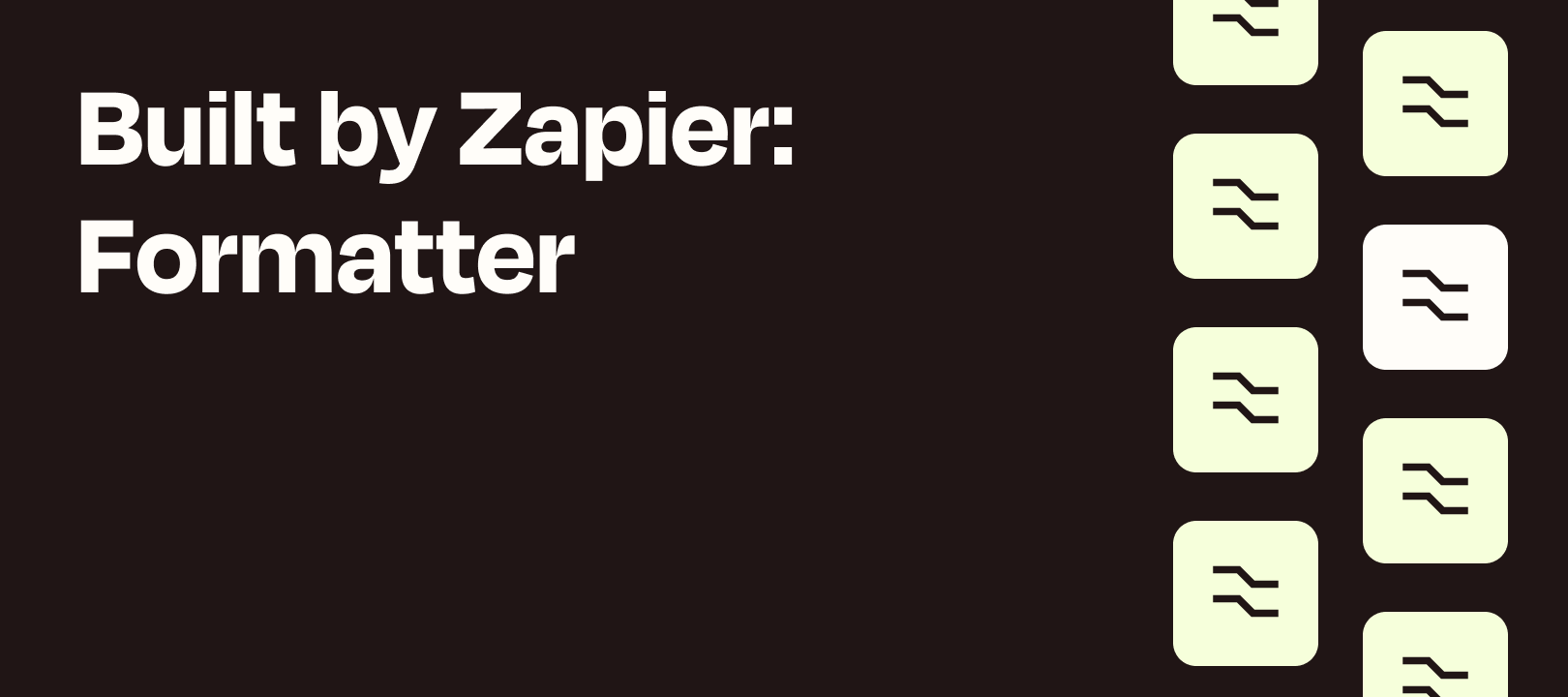# Formatter Features: MEDIAN/AVERAGE and ROUNDUP/ROUNDDOWN in spreadsheet-style formulas

• 0 replies
• 587 viewsUserlevel 7+11
• nicksimard• Community Manager
• 1952 replies

What’s up Zappers? Welcome to the 4th installment of the Formatter Features series (and last of the spreadsheet-style formula posts). If you haven’t done so already, please check out the first three:

## What We’ll be Covering

### MEDIAN / AVERAGE

MEDIAN: Returns the median (the middle value) of a list of numbers
AVERAGE: Returns the average of a list of numbers

### ROUNDDOWN / ROUNDUP

ROUNDDOWN: Returns a number rounded up to a certain number of decimal places
ROUNDUP: Returns a number rounded up to a certain number of decimal places

## When Might You Use Each One?

Let’s take a look at some use cases for each of the above-mentioned functions. That might help you to understand the relevance of these particular spreadsheet-style formulas.

### MEDIAN / AVERAGE

First, let’s look at the difference between the median and average of a set of numbers. You may have seen them used interchangeably, and in some cases the results might be close. Depending what you’re trying to calculate you may want to use one over the other.

MEDIAN: The median of a set of numbers is the number where half of the set’s numbers are lower, and half of the numbers are higher.

AVERAGE: The average of a set of numbers is the result of adding those numbers together and dividing by the number of items in that set.

EXAMPLE:

How much do houses cost in your area?

This is a fairly common question. Given the prices for 9 houses, one would get a very different impression depending on whether you provided the median vs the average.

House prices:

\$450,000 / \$510,000 / \$575,000 / \$685,000 / \$750,000 / \$925,000 / \$1,200,000 / \$1,500,000 / \$2,300,000

Median: \$750,000
Average: \$988,333

I don’t know about you, but \$228,000 (the difference between the median and the average) is a lot of money for me. The reality is that 6 houses are under \$1 million, so \$750,000 is probably a more representative number.

`MEDIAN(number, [number, …])`

IMPORTANT: because each of our numbers should be separated by a comma, this is what happens if we use our house prices as-is:

EXPLANATION: These are all of the comma-separated items ordered from smallest to largest:

000,000,000,000,000,000,000,000,000,1,1,2,200,300,450,500,510,575,685,750,925

Half of the numbers (all of the 000s and the first 1) are smaller than the second 1. The remaining 10 are bigger than 1. That’s why our Formatter step outputs 1.

ALTERNATE APPROACH

We can make all of the numbers the number of thousands (\$1,000,000 would be “1 thousand thousand”, for example):

Practically speaking, we probably wouldn’t bother doing math before and after this step. But for demonstration purposes I wanted to point out what would happen if we used the prices in their original form.

When you might want to return the median:

As discussed, the median is the middle number for a set of numbers that has been arranged from smallest to largest. The median is less affected by outliers and skewed data. As we saw in our house price example, the median gave a more accurate view of what one could expect to pay. Those more expensive outliers skewed things when taking the average.

`AVERAGE(number, [number, …])`

Using the principles we learned above, let’s use the numbers in their adjusted form:

As expected, we get 983.333 (and a whole bunch of other numbers). In the next section I’ll show you how you can combine another function in order to limit the number of decimal places in the output.

When you might want to return the average:

One benefit of using the average is that it includes every value in your number set as part of the calculation. It’s what most people are familiar with, as well. We very often use averages to discuss scores, income, costs, height/weight. If you don’t expect much variation amongst your set of numbers, consider using the average.

OR, you could use both :)

### ROUNDDOWN / ROUNDUP

You can probably guess that ROUNDDOWN and ROUNDUP are related, but it may not be obvious how EVEN is related. Let’s address the ROUNDs first:

`ROUNDUP(number, [places])`

As promised above, I’m going to show you how to combine ROUNDUP with AVERAGE, in order to make this…

988.33333333333334

into this: 988.34

Now let’s run it:

Reasons why you might want to use ROUNDUP:

You’re trying to figure out how many tables you’ll need if they each seat 10 people and you’ve got X number of people coming to your event.

You can’t have 7.4 tables so you’ll want to round up to the next whole number.

`ROUNDDOWN(number, [places])`

As you can imagine, ROUNDDOWN works just like ROUNDUP, except in the opposite direc…

Oh, hmmm...I guess it depends on the numbers!

Reasons why you might want to use ROUNDDOWN:

Example 1: If you charge someone per 15-minute increment (let’s say \$20) and want to make sure that you only ever charge them for whole time periods. You’re nice like thatLet’s say you track minutes like this:

July 12th: 23 minutes / July 14th: 32 minutes / July 15th: 41 minutes / July 16th: 17 minutes

That would give you 7 increments of 15 minutes. Multiply that by \$20 and you get \$140.

## Other Resources

If you’re looking for more information, here are a few places you can look:

1. Community articles
2. Zapier’s help docs (here + here)
3. The other spreadsheet-style Community posts (first, second, third).
4. This video from the first version of Zapier University

## Wrapping Up

Well that’s it for this installment of Formatter Features. Next week, we’ve got the last spreadsheet-style formulas post and after that we’ll be moving on to date formatting :)